• 莫兰指数
2022-07-25 17:19:08

# python求解全局莫兰指数和局部莫兰指数

## 1 数据简介

类别反距离矩阵文件属性值文件
规模520*5201*520
说明无标题行和列无标题行和列

## 2 相关代码

# -*- coding: utf-8 -*-
import matplotlib.pyplot as plt
import numpy as np
import os
import pandas as pd

def moranI(W, X):
'''
W:空间权重矩阵
X:观测值矩阵
'''
#W=W.astype(float)
W = np.array(W)
X = np.array(X)
X = X.reshape(1, -1)
W = W / W.sum(axis=1)  # 归一化
n = W.shape  # 空间单元数
Z = X - X.mean()  # 离差阵
S0 = W.sum()
S1 = 0
for i in range(n):
for j in range(n):
S1 += 0.5 * (W[i, j] + W[j, i]) ** 2
S2 = 0
for i in range(n):
S2 += (W[i, :].sum() + W[:, i].sum()) ** 2
# 全局moran指数
I = np.dot(Z, W)
I = np.dot(I, Z.T)
I = n / S0 * I / np.dot(Z, Z.T)
# 在正太分布假设下的检验数
EI_N = -1 / (n - 1)
VARI_N = (n ** 2 * S1 - n * S2 + 3 * S0 ** 2) / (S0 ** 2 * (n ** 2 - 1)) - EI_N ** 2
ZI_N = (I - EI_N) / (VARI_N ** 0.5)
# 在随机分布假设下检验数
EI_R = -1 / (n - 1)
b2 = 0
for i in range(n):
b2 += n * Z[0, i] ** 4
b2 = b2 / ((Z * Z).sum() ** 2)
VARI_R = n * ((n ** 2 - 3 * n + 3) * S1 - n * S2 + 3 * S0 ** 2) - b2 * (
(n ** 2 - n) * S1 - 2 * n * S2 + 6 * S0 ** 2)
VARI_R = VARI_R / (S0 ** 2 * (n - 1) * (n - 2) * (n - 3)) - EI_R ** 2
ZI_R = (I - EI_R) / (VARI_R ** 0.5)
# 计算局部moran指数
Ii = list()
for i in range(n):
Ii_ = n * Z[0, i]
Ii__ = 0
for j in range(n):
Ii__ += W[i, j] * Z[0, j]
Ii_ = Ii_ * Ii__ / ((Z * Z).sum())
Ii.append(Ii_)
Ii = np.array(Ii)
# 局部检验数
ZIi = list()
EIi = Ii.mean()
VARIi = Ii.var()
for i in range(n):
ZIi_ = (Ii[i] - EIi) / (VARIi ** 0.5)
ZIi.append(ZIi_)
ZIi = np.array(ZIi)
'''
# moran散点图
# 用来正常显示中文标签
plt.rcParams['font.sans-serif'] = ['SimHei']
# 用来正常显示负号
plt.rcParams['axes.unicode_minus'] = False
fig = plt.figure()
ax.spines['top'].set_color('none')
ax.spines['right'].set_color('none')
ax.xaxis.set_ticks_position('bottom')
ax.spines['bottom'].set_position(('data', 0))
ax.yaxis.set_ticks_position('left')
ax.spines['left'].set_position(('data', 0))
WZ = np.dot(Z, W)
ax.scatter(Z, WZ, c='k')
x1 = range(int(Z.min()), int(Z.max() + 1))
y1 = range(int(Z.min()), int(Z.max() + 1))
ax.plot(x1, y1, 'k--', label='x=y')
x2 = list(range(int(Z.min()), int(Z.max() + 1)))
y2 = np.array(x2) * I
ax.plot(x2, y2, 'k-', label='I*x=y')
ax.legend(loc='upper right')
imgPath = os.path.join(os.getcwd(), '莫兰散点图.png')
ax.set_title('莫兰散点图')
fig.savefig(imgPath)
'''
return {
'I': {'value': I[0, 0], 'desc': '全局moran指数'},
'ZI_N': {'value': ZI_N[0, 0], 'desc': '正太分布假设下检验数'},
'ZI_R': {'value': ZI_R[0, 0], 'desc': '随机分布假设下检验数'},
'Ii': {'value': Ii, 'desc': '局部moran指数'},
'ZIi': {'value': ZIi, 'desc': '局部检验数'},
#'img': {'path': imgPath, 'desc': '莫兰散点图路径'}
}

if __name__ == "__main__":

w=np.array(df)
x = np.array(df1)
print(len(data),len(x))
result=moranI(w, x)
print(result['I'],result['ZI_N'])



运行结果如下：

{'value': 0.18758732033404646, 'desc': '全局moran指数'} {'value': 31.11189591476361, 'desc': '正太分布假设下检验数'}



## 3 matlab相关方法

matlab求解全局莫兰指数：
①文件名为moransi.m
②直接调用传入参数即可



function global_moran_test(x0,w)

row = size(x0,2);
moran.mean = zeros(row,1);
moran.num = zeros(row,1);
moran.stdev = zeros(row,1);
moran.index = zeros(row,1);
moran.z = zeros(row,1);
moran.p = zeros(row,1);

moran.globalresult = zeros(row,3);

for r = 1 : 1 : row
x = x0(:,r);
moran.mean(r) = mean(x);
moran.num(r) = size(x,1);
moran.stdev(r) = std(x);

z_x = (x - moran.mean(r)) / moran.stdev(r);
sum_wij = 0;
s = 0;
s1 = 0;
s2 = 0;
m2 = 0;
m4 = 0;
for a = 1 : 1 : moran.num(r)
w_i = 0;
w_j = 0;
m2 = m2 + (x(a,1) - moran.mean(r))^2;
m4 = m4 + (x(a,1) - moran.mean(r))^4;
for b = 1 : 1 : moran.num(r)
sum_wij = sum_wij + (w(a,b) * z_x(a,1) * z_x(b,1));
s = s + w(a,b);
s1 = s1 + (w(a,b) + w(b,a))^2;
w_i = w_i + w(a,b);
w_j = w_j + w(b,a);
end
s2 = s2 + (w_i + w_j)^2;
end
m2 = m2 / moran.num(r);
m4 = m4 / moran.num(r);
b2 = m4 / (m2^2);
sum_i2 = 0;
for a = 1 : 1 : moran.num(r)
sum_i2 = sum_i2 + (z_x(a,1) * z_x(a,1));
end
moran.index(r) = (moran.num(r) * sum_wij) / (s * sum_i2);

n = moran.num(r);
temp_1 = n * (n^2 - 3 * n + 3) * s1 - (n * s2) + (3 * s^2);
temp_2 = b2 * ((n^2 - n) * s1 - (2 * n * s2) + (6 * s^2));
den = (n - 1) * (n - 2) * (n - 3) * (s^2);
sd = (temp_1 - temp_2) / den - (1 / (n-1))^2;
sd = sqrt(sd);

e = -1 / (n - 1);

moran.z(r) = (moran.index(r) - e) / sd;

moran.p(r) = 1 - normcdf(moran.z(r));
end

moran.globalresult(:,1) = moran.index;
moran.globalresult(:,2) = moran.z;
moran.globalresult(:,3) = moran.p;

fprintf('%6s %12s %18s %24s\r\n','t','Moran','z','p');
for i = 1 : 1 : row
fprintf('%6.3f %12.3f %18.3f %24.3\n',i,moran.index(i),moran.z(i),moran.p(i));
end
endpython numpy 开发语言
更多相关内容
• 用stata算莫兰指数的相关命令，空间计量需要用的。
• matlab做莫兰指数的代码SSLB-示例 此存储库包含用于重现论文“Spike-and-Slab Lasso Biclustering”（Moran、Rockova 和 George，2020 年）中的结果的 R 代码。 SSLB R 包在 Github 存储库中可用： ...
• ## 莫兰指数

千次阅读 2021-05-27 14:46:16
莫兰指数分为全局莫兰指数（Global Moran's I）和局部莫兰指数（Local Moran's I），前者是Patrick Alfred Pierce Moran开发的空间自相关的度量；后者是美国亚利桑那州立大学地理与规划学院院长Luc Anselin 教授在...

​自己理解着瞎写，主要是6个小部分

一、莫兰指数

二、莫兰指数、P值、Z值

三、零假设与置信度

四、空间关系概念化

五、距离法

六、标准化

一、莫兰指数

莫兰指数分为全局莫兰指数（Global Moran's I）和局部莫兰指数（Local Moran's I），前者是Patrick Alfred Pierce Moran开发的空间自相关的度量；后者是美国亚利桑那州立大学地理与规划学院院长Luc Anselin 教授在1995年提出的。在Arcgis里分别是“空间自相关”与“聚类和异常值分析”工具。

通常情况，先做一个地区的全局莫兰指数，全局指数告诉我们空间是否出现了集聚或异常值，但并没有告诉我们在哪里出现，回答有或无。

如果全局有自相关出现，接着做局部自相关，局部Moran'I会告诉我们哪里出现了异常值或者哪里出现了集聚。

二、莫兰指数、P值、Z值的解读

Moran's I的范围在 -1.0 与 +1.0 之间。

Moran's I大于0时，表示数据呈现空间正相关，其值越大空间相关性越明显；Moran's I小于0时，表示数据呈现空间负相关，其值越小空间差异越大；Moran's I为0时，空间呈随机性。

解读莫兰指数的时候，需要有P值和Z得分来判定。他们两个要结合在一起看的。如下表与下图。

P值：（P-Value，Probability，Pr），概率的意思。当p很小时，意味着所观测到的空间模式不太可能产生于随机过程（小概率事件），因此可以拒绝零假设。

Z得分：（standard score），标准分数。记住标准差能反映一个数据集的离散程度，就可以了。

 z 得分（标准差） p 值（概率） 置信度 < -1.65 或> +1.65 < 0.10 90% < -1.96 或> +1.96 < 0.05 95% < -2.58 或 > +2.58 < 0.01 99%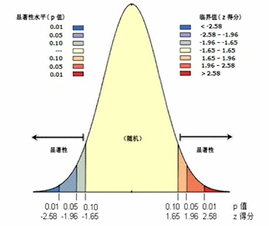先看图中，分为三部分，中间黄色部分为随机分布，右侧为集聚分布，左侧为离散分布。

下面有P值与Z得分两行数值。再结合表格看

假如P值＜0.01，且Z得分＞2.58，那么就落在图的最最最右边红色的区域，我们可以说有99%的把握要素是集聚分布的。对应的，如果P值＜0.01，且Z得分<-2.58，那么就落在图的最最最左边蓝色的区域，我们可以说有99%的把握要素是离散分布的。

假如P值＜0.01，但Z得分<2.58，那么就表示不可以拒绝零假设，实验是失败的。这里面又涉及到统计上以下两个概念。

三、零假设与置信度

1、零假设：官方的解释是指进行统计检验时预先建立的假设。这个“零假设”的设立是为了去否定它的，空间统计中的零假设是指“我想统计的空间要素是随机分布的”，要去做的也就是去证明要素不是随机分布的，是呈现聚类或者离散分布的。

2、置信度：比如我这个实验结论有95%的置信度，意义就是我有95%的把握拒绝零假设，证明零假设是错误的，是可以实现这个结果。置信区间是保证这个置信度的变量或参数的区间范围。区间越大猜中概率越大。

四、空间关系概念化

在操作窗口，会让选择空间关系概念化，一共有七种，了解了一下

1、INVERSE_DISTANCE（反距离）：与远处的要素相比，附近的邻近要素对目标要素的计算的影响要大一些。

2、INVERSE_DISTANCE_SQUARED（反距离平方）：与第一种相似，但它对距离反应更为敏感。所以这两种方法区别就在于附近邻近要素对目标要素的计算的影响有多大，特别大就第二种。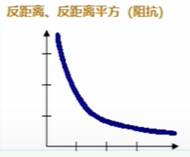如果横坐标代表距离，纵轴代表影响力，那么随着距离增加，影响力就变得越来越小。这就是反距离。

3、 FIXED_DISTANCE_BAND（距离范围影响）：将对邻近要素环境中的每个要素进行分析。在指定临界距离（距离范围或距离阈值）内的邻近要素将分配有值为 1 的权重，并对目标要素的计算产生重大影响。在指定临界距离外的邻近要素将分配值为零的权重，并且不会对目标要素的计算产生任何影响。其实就是在一定范围内的临近要素对目标要素影响力是一样的，不存在随距离增加而减小。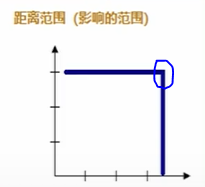横轴代表距离，纵轴代表影响力，在到达蓝圈圈这个点之前，影响力不会衰减，都是一样的。过了这个点就不会产生影响。

4、ZONE_OF_INDIFFERENCE（无差别的区域）：在目标要素的指定临界距离（距离范围或距离阈值）内的要素将分配有值为1的权重，并且会影响目标要素的计算。一旦超出该临界距离，权重（以及邻近要素对目标要素计算的影响）就会随距离的增加而减小。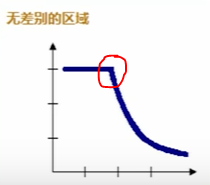可以看做是“反距离”与“距离范围”的结合，在红圈圈之前影响力都是一样的，超过这个红圈圈，影响力便随着距离增加而减小。

5、 CONTIGUITY_EDGES_ONLY—只有共用边界或重叠的相邻面要素会影响目标面要素的计算。

6、CONTIGUITY_EDGES_CORNERS—共享边界、结点或重叠的面要素会影响目标面要素的计算。可以看到出现了corners角落这个词。

看下面图，我要研究A区域受哪些区域影响，假如我选取了ONLY，那么B和D与A是有共用边的，而C没有共边，所以C是没影响的。假如我选择CORNERS，那么BCD都有影响，因为他们共享了角。在GeoDa里有Rook和Queen，对应的就是only与corners。五、距离法

指定计算每个要素与邻近要素之间的距离的方式。在操作窗口，也会让选择距离法，有两种。

1、欧氏距离—两点间的直线距离。

2、曼哈顿距离—沿垂直轴度量的两点间的距离（城市街区）；计算方法是对两点的 x 和 y 坐标的差值（绝对值）求和。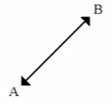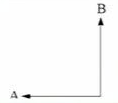直线距离                                  两点之间的垂直距离

六、标准化

最后还有一个标准化记择得选ROW。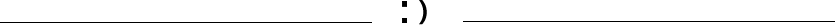更多欢迎关注【一点规划】展开全文arcgis
• 1.用excel自带公式自动计算莫兰指数，局部莫兰指数，以及二者的校验。 2.附超详细的计算步骤说明，好用，易懂，也便于对公式的更进一步的理解和检验。 3.能很方便的绘制莫兰散点图。 软件买不起，也不好用，走了好多...
• Stata做空间杜宾模型、莫兰指数等操作_Hellolijunshy的博客-CSDN博客_stata做空间杜宾模型实例.mht
• 那么空间分布模式，也可以有这种能力，就是通过一个指数来进行整体描述，这个指数，就是我们今天要说的莫兰指数（Moran‘s I）。 啥叫“指数”呢？我们在日常生活中，实际上也经常听到各种“指数”，比如新闻里面...
• 用python实现莫兰指数计算
import esda
import pandas as pd
import geopandas as gpd
from geopandas import GeoDataFrame
import libpysal as lps
import numpy as np
import matplotlib.pyplot as plt
from shapely.geometry import Point
import contextily as ctx
from pylab import figure, scatter, show
import warnings
warnings.filterwarnings('ignore')
%matplotlib inline


关于geopandas和contextily库的安装，参考我的另一篇博客https://www.jianshu.com/p/a1496e316bb4

#### 读取数据

一个是地图信息，一个是需要研究的指标。

# 除中华人民共和国.shp外其余三个文件必须也在，只有一个则读取出错
# 中华人民共和国.dbf
# 中华人民共和国.prj
# 中华人民共和国.shx


#### 构建邻接矩阵

wq = lps.weights.Queen.from_dataframe(gdf_data) #使用Quuen式邻接矩阵
wq.transform = 'r' #标准化矩阵
# 两个省没有直接相邻的省（34行数据不清楚是什么，下载地图shp文件中自带的）
print(gdf_data.loc[[20,31],'name'])
'''
('WARNING: ', 20, ' is an island (no neighbors)')
('WARNING: ', 31, ' is an island (no neighbors)')
('WARNING: ', 34, ' is an island (no neighbors)')
20    海南省
31    台湾省
Name: name, dtype: object
'''

centroids = gdf_data.geometry.centroid # 计算多边形几何中心
# 本图将邻接矩阵覆盖在整个地图上，如果只需要邻接矩阵，删除ax = gdf_data.plot(figsize=(10, 10),cmap="Blues")
ax = gdf_data.plot(figsize=(10, 10),cmap="Blues")
plt.plot(centroids.x, centroids.y, '.')
for k, neighs in wq.neighbors.items():
origin = centroids[k]
for neigh in neighs:
segment = centroids[[k,neigh]]
plt.plot(segment.x, segment.y, '-')
plt.title('Queen Neighbor Graph')
plt.axis('off')
plt.show()
plt.savefig("save/{}.jpg".format('wq'),dpi=300) # 图片模糊问题，需要指定图片的dpi大小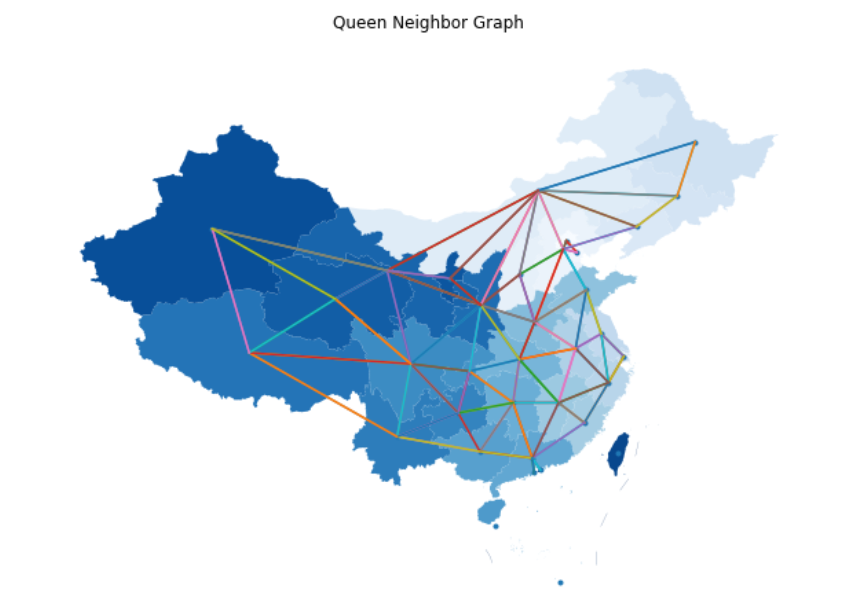#### 批量计算2010-2020年的全局莫兰指数

合并数据，自动去掉不研究的数据（不带指标的省份）

# 实际数据只研究30个省份，不包含香港澳门，台湾和西藏
gdf_drop = gdf_data.merge(feature_df,left_on='name',right_on='地区')
wq = lps.weights.Queen.from_dataframe(gdf_drop)
wq.transform = 'r' # 标准化矩阵
centroids = gdf_drop.geometry.centroid # 计算多边形几何中心
wr = lps.weights.Rook.from_dataframe(gdf_drop)# 使用Rook式邻接矩阵

Moran_I_list = []
random_Z_list = []
random_Zp_list = []
norm_Z_list = []
norm_Zp_list = []

for i in range(12, 23): # 按照列名位置
y_name = gdf_drop.columns[i]
y = gdf_drop[y_name]
mi = esda.moran.Moran(y, wq)

Moran_I_list.append(mi.I)
random_Z_list.append(mi.z_rand)
random_Zp_list.append(mi.p_rand)
norm_Z_list.append(mi.z_norm)
norm_Zp_list.append(mi.p_norm)

Moran_I_df = pd.DataFrame()
Moran_I_df['year'], Moran_I_df['Moran_I'], Moran_I_df['random_Z'], Moran_I_df['random_p'], Moran_I_df['norm_Z'], Moran_I_df['norm_p'] = \
(range(2010, 2021), Moran_I_list, random_Z_list, random_Zp_list, norm_Z_list, norm_Zp_list)

print(Moran_I_df)
'''
year   Moran_I  random_Z  random_p    norm_Z    norm_p
0   2010  0.4xxxxx  4.xxxxx   0.0xxxxx    4.1xxxxx   0.0xxxxx
1   2011  0.4xxxxx  3.xxxxx   0.0xxxxx    3.7xxxxx   0.0xxxxx
2   2012  0.4xxxxx  3.xxxxx   0.0xxxxx    3.8xxxxx   0.0xxxxx
3   2013  0.4xxxxx  3.xxxxx   0.0xxxxx    3.9xxxxx   0.0xxxxx
4   2014  0.4xxxxx  4.xxxxx   0.0xxxxx    4.0xxxxx   0.0xxxxx
（省略）
'''


#### 以2020年为例展示计算的莫兰指数和检验结果

y = gdf_drop["2020y"]
mi = esda.moran.Moran(y, wq)

print("Moran's I 值为：",mi.I)
print("随机分布假设下Z检验值为：",mi.z_rand)
print("随机分布假设下Z检验的P值为：",mi.p_rand)
print("正态分布假设下Z检验值为：",mi.z_norm)
print("正态分布假设下Z检验的P值为：",mi.p_norm)
'''
Moran's I 值为： 0.4542425813757728
随机分布假设下Z检验值为： 4.xxxxxx
随机分布假设下Z检验的P值为： 6.xxxxxxe-05
正态分布假设下Z检验值为： 4.xxxxxx
正态分布假设下Z检验的P值为： 5.xxxxxx-05
'''


#### 绘制2020年的莫兰散点图

from splot.esda import plot_moran
fig, ax = plot_moran(mi, zstandard=True, figsize=(11,5),aspect_equal=False)
fig.show()
fig.savefig("save/{}.jpg".format('清洁低碳全局莫兰散点图'),dpi=300) # 图片模糊问题，需要指定图片的dpi大小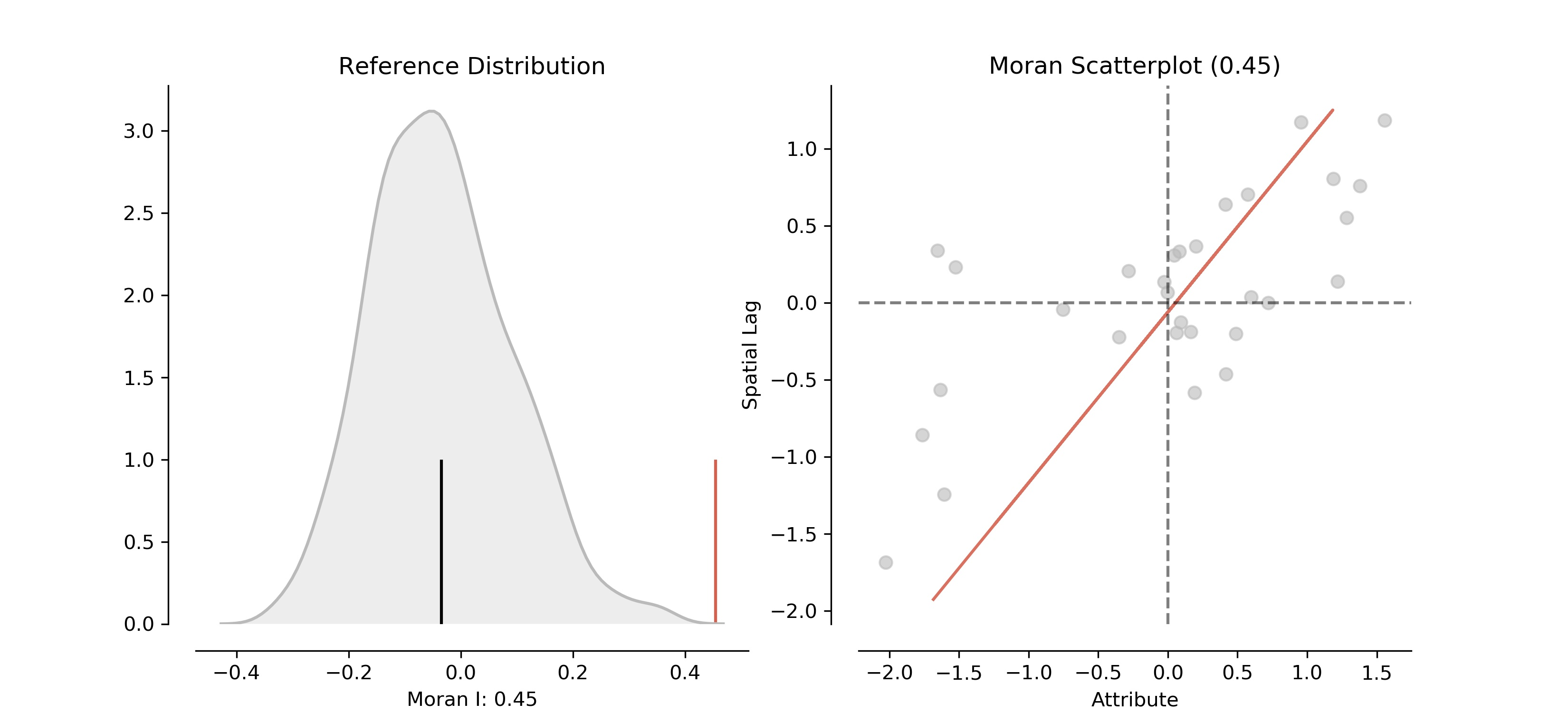#### 2020年全局莫兰指数检验通过，继续做局部莫兰指数(全局不通过，不用做局部)

from splot.esda import moran_scatterplot
from esda.moran import Moran_Local
from splot.esda import lisa_cluster
from splot.esda import plot_local_autocorrelation

y = gdf_drop['2020y'].values
w = lps.weights.distance.Kernel.from_dataframe(gdf_drop, fixed=False, k=15)
w.transform = 'r'
moran_loc = Moran_Local(y, w)

# 局部莫兰指数
loc_moran_df = pd.DataFrame({'name':gdf_drop.name, 'loc_moran':moran_loc.Is}) # moran_loc.Is是各省的局部莫兰指数
print(loc_moran_df)
'''
name  loc_moran
0        北京市  -0.033960
1        天津市   0.319125
2        河北省  -0.086364
3        山西省   0.000686
4     内蒙古自治区   0.068562
5        辽宁省  -0.063257
6        吉林省   0.002306
7       黑龙江省  -0.012012
8        上海市   0.001538
9        江苏省   0.025877
10       浙江省   0.307162
(省略)
'''


#### 局部莫兰散点图

fig, ax = moran_scatterplot(moran_loc, p=0.1,aspect_equal=True)
#ax.set_xlabel('FSTGDPRATE')
#ax.set_ylabel('Spatial Lag of FSTGDPRATE')
#plt.show()
fig.savefig("save/{}.jpg".format('清洁低碳局部莫兰散点图'),dpi=300) # 图片模糊问题，需要指定图片的dpi大小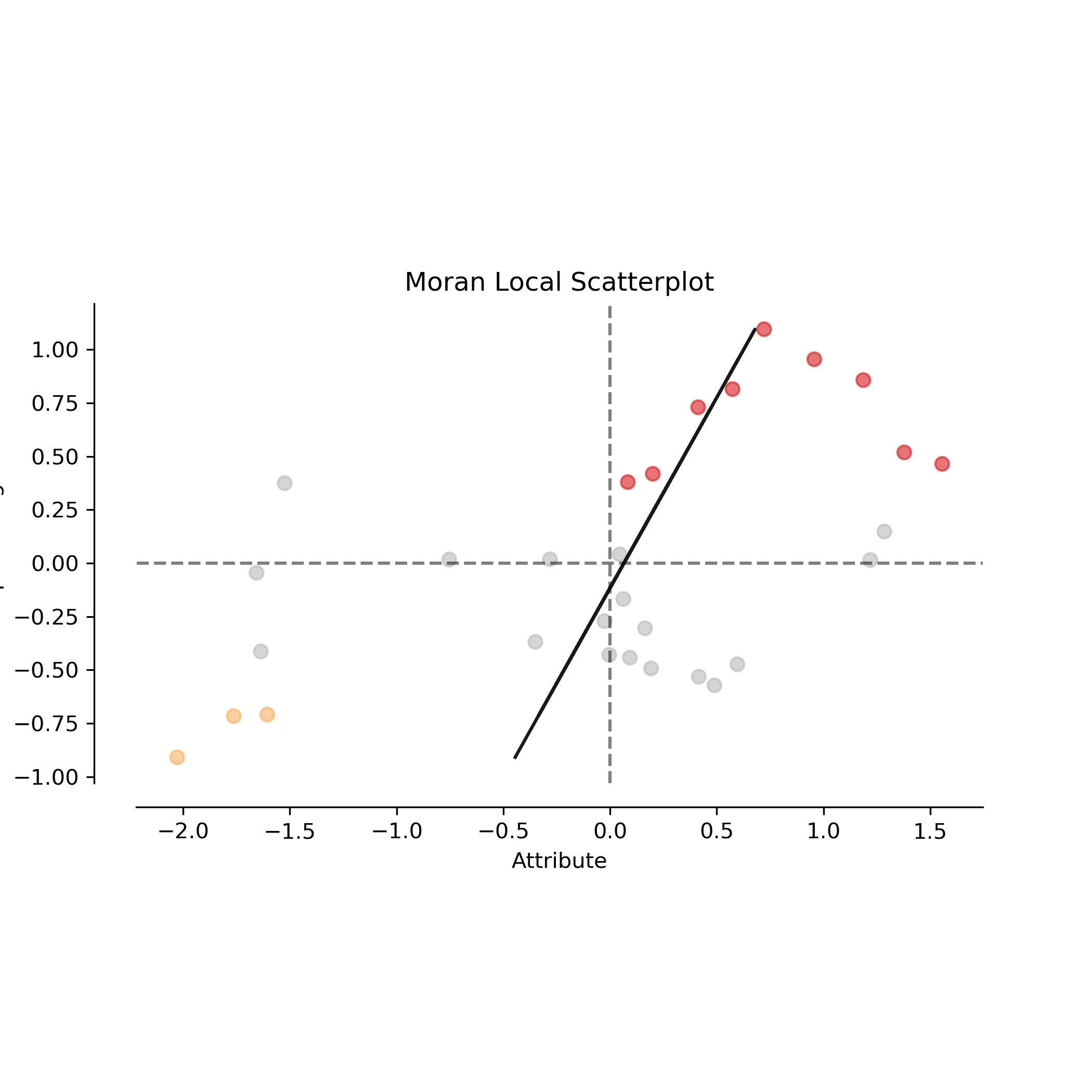#### 空间分布图(不展示组合图)

**强调：**没有计算西藏地区，所以中国大陆地图中缺失西藏地区

fig, ax = lisa_cluster(moran_loc, gdf_drop, p=0.1, figsize = (9,9))
fig.savefig("save/{}.jpg".format('清洁低碳聚集区的空间分布图'),dpi=300) # 图片模糊问题，需要指定图片的dpi大小

#绘制组合图(不展示组合图)
#fig, ax = plot_local_autocorrelation(moran_loc, gdf_drop, '2020y',p=0.1,figsize = (27,6))
#fig.savefig("save/{}.jpg".format('清洁低碳组合图'),dpi=300) # 图片模糊问题，需要指定图片的dpi大小参考：

### 实操问题

做完全局莫兰指数后，计算局部莫兰指数的函数 Moran_Local 一直出错，其中提到 numba 库的问题。我查询报错，发现有个提升 numba 版本的说法，然后我升级到最新版本。随后直接导致 esda 库和 libpysal 库导入失败，导致全局莫兰指数部分无法运行。随后我卸载了numba，esda 和 libpysal，再次安装 numba，esda 和 libpysal，依然无法导入 esda 和 libpysal。尝试多种方法后，我再一次卸载了 numba，esda 和 libpysal，然后只安装 esda 库，神奇的是我可以导入esda，甚至可以直接导入 libpysal，连之前出错的 Moran_Local 函数也可以运行了。我推测是Moran_Local 依赖的 numba 库版本和我的 numba 库版本不一致，在我将 numba 库版本升级成最新版时，又与 esda 和 libpysal 不匹配。最后我删除 numba 后，只安装最新的 esda，会自己下载合适的 numba，然后就可以运行 Moran_Local 函数（esda.moran.Moran_Local）。
此处展示我的环境：

• Python 3.6.5
• numba 0.38.0
• esda 2.4.1
• libpysal 4.5.0
展开全文python numpy
• 莫兰指数分为全局莫兰指数和局部莫兰指数。 // 全局和局部 // 其中，全局型的功能在于描述某现象的整体分布状况，判断此现象在空间是否有聚集特性存在，但其并不能确切地指出聚集在哪些地区。而局部型可以进一步...空间自相关：是指一些变量在同一个分布区内的观测数据之间潜在的相互依赖性。其中，自相关中的“自”表示当你进行相关性观察统计量，是来源于不同对象的同一属性。Tobler(1970)曾指出“地理学第一定律：任何东西与别的东西之间都是相关的，但近处的东西比远处的东西相关性更强”。

空间自相关统计量是用于度量地理数据(geographic data)的一个基本性质：某位置上的数据与其他位置上的数据间的相互依赖程度。

在地理统计学科中应用较多，现已有多种指数可以使用，但最主要的有两种指数，即Moran的I指数Geary的C指数，也就是我们常说的莫兰指数和G统计量。

今天我们就先了解一下度量空间相关性的一个重要指标之一的莫兰指数

莫兰指数分为全局莫兰指数局部莫兰指数

// 全局和局部 //

其中，全局型的功能在于描述某现象的整体分布状况，判断此现象在空间是否有聚集特性存在，但其并不能确切地指出聚集在哪些地区。而局部型可以进一步告诉我们哪个位置出现了异常值、哪个位置出现了集聚现象。它可以判断一座座居民楼它们是聚集在一块还是离散分布在各处。

// 值的分布 //莫兰指数是一个有理数，通过方差归一化操作之后，其值将分布在[-1,1]之间，用来判别空间是否存在自相关。当值大于0时，表示数据呈现空间正相关，其值越大空间相关性越明显。当值小于0时，表示数据呈现空间负相关，其值越小空间差异越大。当值等于0时，空间呈随机性。

// 在Arcmap中的位置 //在Arcmap中进行空间自相关分析时，可以打开工具箱【空间统计工具】下面的对应的位置，分析之后会得到下方的结果。解读这个结果的时候，大家可以关注其P值和Z值的得分即可。当P值小于0.05(通过95%置信度检验)，且Z得分超过临界值1.65(拒绝零假设设定的阈值)；Z分数为负号，且通过显著性检验，比如而上图中的P值大于了0.05的95%的置信度，Z值也没有超过1.65，所以说这个数据就偏向随机了。

// 相关文章 //

《基于Moran统计量的空间自相关理论发展和方法改进》

陈彦光 北京大学城市与环境学院文章链接：

提取码：

plcf展开全文• ## Stata计算莫兰指数基本步骤

万次阅读 多人点赞 2021-11-27 10:41:43
之前的博客有介绍过R和Geoda计算莫兰指数的方法，考虑到有时候我们需要自定义空间权重矩阵来计算莫兰指数，那以上两种方法显得有点复杂。所以，今天来分享Stata计算莫兰指数的方法~ 目录一、数据准备1.1 数据导入1.2...空间计量分析
• 全局莫兰指数莫兰指数） 和 局部莫兰 是用于计算Spatial autocorrelation（空间自相关性）。 所谓空间自相关性，是指特征信号是否与空间相邻位置存在相关性。 （维基百科：wiki） 大白话翻译就是，二维空间中，...python 数据挖掘
• 全局莫兰指数主要是观察指标的整体的聚集效应，莫兰指数大于0呈现空间正相关，小于0呈现空间负相关。计算公式如下： 公式截图来自我的论文初稿，自己总结的，可能存在一些错误，具体的可以自行查阅权威论文。 局部...arcgis
• 莫兰指数（Moran’s I）是研究变量在同一个分布区内的观测数据之间潜在的相互依赖性的一个重要研究指标，在本文中，我们将探讨局域（Anselin Local Moran I）与全域两种莫兰指数（Moran I）计算在Arcgis中的实现。...gis
• [TOC]程序简介计算省的pm2.5平均值作为观测矩阵，省会的距离的倒数作为空间权重矩阵，计算全局莫兰指数为0.49，显著性检验p值为3.75>1.96，得出中国地区的pm2.5存在空间正相关输入：中国各地区pm2.5值 输出：莫兰...
• 在上篇中，我们详细地阐述了全局莫兰指数(Global Moran’I)的含义以及具体的软件实操方法。今天，就来进一步地说明局部莫兰指数(Local Moran’I)的含义与计算。         ...
• 目录 一、公式说明 二、深入理解 三、Moran'I指数检验 四、R和Geoda计算莫兰指数 （1）R （2）Geoda 一、公式说明 在全局相关分析中，最常用的统计量就是Global Moran’I(全局莫兰指数)，它主要是用来描述所有的...r语言
• 莫兰指数的p值大于0.1可能是什么情况，该如何解决，求大神！！！httpx
•matlab 开发语言
• 提出者为澳大利亚统计学家帕特里克·阿尔弗雷德·皮尔斯·莫兰（Patrick Alfred Pierce Moran）。 Moran PAP. The interpretation of statistical maps[J]. Journal of the Royal Statistical Society B , 1948,(37...arcgis
• Moran指数分为全局和局部，两者都是用来分析空间相关性的一个指标，全局莫兰指数用来分析有没有空间自相关性存在，而局部莫兰指数用来探测异常值或者集聚出现的范围和位置。在之前的论文中，有个问题困扰了我很久，...
• 资源名：morans_i_莫兰指数_matlab 资源类型：matlab项目全套源码 源码说明： 全部项目源码都是经过测试校正后百分百成功运行的，如果您下载后不能运行可联系我进行指导或者更换。 适合人群：新手及有一定经验的开发...
• ## 空间自相关—莫兰指数

千次阅读 多人点赞 2021-07-07 09:44:06
莫兰指数分为全局莫兰指数（Global Moran's I）和局部莫兰指数（Local Moran's I），前者是Patrick Alfred Pierce Moran开发的空间自相关的度量；后者是美国亚利桑那州立大学地理与规划学院院长Luc Anselin 教授在...arcgis
• 莫兰指数-计算步骤、数据&事件研究Stata代码python
• Geoda软件 一、数据处理 二、Geoda--莫兰指数画图 一、数据处理 1、数据处理 我要做的操作是对各套数据源进行莫兰指数计算，看Moran’s散点图、显著性地图、聚类地图相关内容。 要画出上述所说的内容，就要把数据给...聚类 机器学习 数据挖掘
• 莫兰指数为正，而空间杜宾模型里的rho为负值，这是为什么呀
• 那么这个所谓的莫兰指数，就是用来衡量空间自相关的程度的一个综合性评价——特指全局莫兰指数。 关于空间自相关，我以前也写过一篇文章，大家有兴趣就去翻历史文章吧，这里仅作简单的回顾。其实空间自相关要是把...
• CSDN的被爬虫专用声明：虾...首先我们用常规的方式演示一下在GeoDa里面如何做莫兰指数： 打开GeoDa，打开我们需要分析的数据，比如还是中国的人口GDP的shape file（数据获取在虾神的gitee&github上面，公众号发送空间关系 空间分析 GIS
• 上一篇简单说了一下莫兰指数的计算原理和计算公式，如果是学生或者基础研究者，鼓励好好的学习一下手算或者编程计算，所谓的基础不牢，地动山摇……但是对于工程界...
• 资源名：计算莫兰指数_用于评估空间影响关系_空间分析_matlab 资源类型：matlab项目全套源码 源码说明： 全部项目源码都是经过测试校正后百分百成功运行的，如果您下载后不能运行可联系我进行指导或者更换。 适合......